# Bayesian Weight Loss

In a previous post, I discussed the concept of linear regressions in the realm of Bayesian statistics. I will do something similar in this post but we’ll change the topic a little bit. We’ll go from finance to weight loss.

# Introduction

I have recently decided to start being more healthy in my life (about time). However, if I wanted to make a change in my life, it meant I was going to have to measure those changes to make sure I’m going in the right direction. In terms of my weight, I decided to purchase a Withings Scale to constantly track my weight and other important metrics.

As I started weighting myself, I did so twice a week, on Mondays and Thursdays. I started to realised there was quite a big variation in weight from those two data points and suspected there could be some weekly seasonality (I confess I might eat a bit more during the weekends that I do during the week). To properly understand this seasonality, I decided to weigh myself every single day, at the same time of the day (in the morning before my shower).

The problem was that it became harder to know if I was losing weight or not. Some days it would go up some other days it would go down, but it was hard to understand the pattern and the trends.

Being the data-loving person that I am, I decided to look at the data more carefully.

# Data

My Withings scale is synced with my Apple Health, so it was incredibly easy to just download all of my data to start my analysis. I imported the XML data in Python selected the weight data from all the metrics collected from Apple and started to explore it.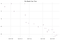Scatter plot of my weight recordings over time

I find the variations in my weight over time quite interesting, but at this point, it seems quite obvious that I am indeed losing weight. Let’s keep exploring.

The relation looks quite linear to me and I thing a lineal model would make a reasonable approximation. Besides, a linear model would offer an extremely simple interpretation. I would just look at the β coefficient. This coefficient determines the slope of the regression line, which translates into my change in weight over time. If the slope is negative (i.e. β is negative) then I am losing weight over time. If β ends up being positive it means I am actually gaining weight and if it’s zero then I am neither losing nor gaining weight.

A priori, it is quite likely that β will be negative, indicating a weight-losing trend.

# OLS

I will start with an Ordinary Least Squares model, to have a benchmark and because it’s super easy to implement. All I have to do is regress my weight over time: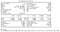Summary of results from OLS regression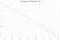OLS regression line

From these results, it seems my hypothesis was correct. I seem to be losing weight. However, if you read any of my other posts, you’ll know that this is not enough for me. I want to understand how likely it actually is that I am losing weight. I also want to be able to estimate my current weight and understand the probability behind that estimation.

# Bayesian Regression

To be able to answer these questions, we need to switch to a Bayesian model. If you want to read more about Bayesian linear regressions, check my previous post: Bayesian CAPM Beta Estimation.

In this case, I will use quite uninformative priors, but with (in my opinion) quite reasonable logic. For my alpha parameter which will be a sort of average weight parameter, I will use a normal distribution with quite a huge sigma to reflect my lack of knowledge about its actual value, but a mean to reflect a reasonable guess (70kg). I will also use a normal distribution for my beta and will centre it at 0 to be able to capture both weight loss or gains. Now I can build the model using PyMC3:

After checking traces for convergence and diagnostics, I can start analysing the posterior distribution. We can start looking at the distribution of my beta parameter: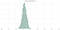Posterior distribution of β

This looks good! It seems I am really losing weight and my posterior distribution assigns no probability to the possibility of me NOT having a weight-loss trend. Let’s look at the actual regression: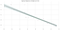Bayesian regression line

The regression also looks good, we can see that it’s starting to lose certainty as it becomes more unsure about the parameters closer to the current date, but it is pretty certain that I lost weight. Talking about my weight, we can use the estimates for beta and alpha to try to estimate my current weight. Or even better, generate a distribution for my current weight: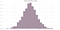Posterior distribution of my weight

That’s interesting, we can see that uncertainty we saw in the regression line being reflected in this distribution. We are, in fact, quite unsure about my current weight. However, we can use Bayesian 95% credible intervals to understand this better.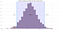Posterior distribution of my weight with 95% credible intervals

It seems my weight is most likely (95% probability) between 67.6kg and 68.1kg. We can even use this distribution to find some probabilities. For example, it seems I’m likely to weight less than 68kg, there’s only an 8.3% probability that I weight more than that:

# Conclusion

This post was yet another example of how to build linear regressions using Bayesian statistics. Hopefully, this serves as proof of how useful Bayesian statistics can be and reflects the power behind having an entire distribution of your parameters. In essence, we are able to quantify our uncertainty instead of just hiding it behind a single number estimation.

As far as my health goes, I’ll start keeping track of my beta from now on to make sure I am still keeping myself healthy even if I’m losing weight. This is hugely useful as losing weight too fast can have detrimental results (source). At the same time, my Withings scale records other really nice metrics which I’ll probably build some other Bayesian models to try to understand a little better.

## More from Juan Gesino

Head of Data @ Sirena